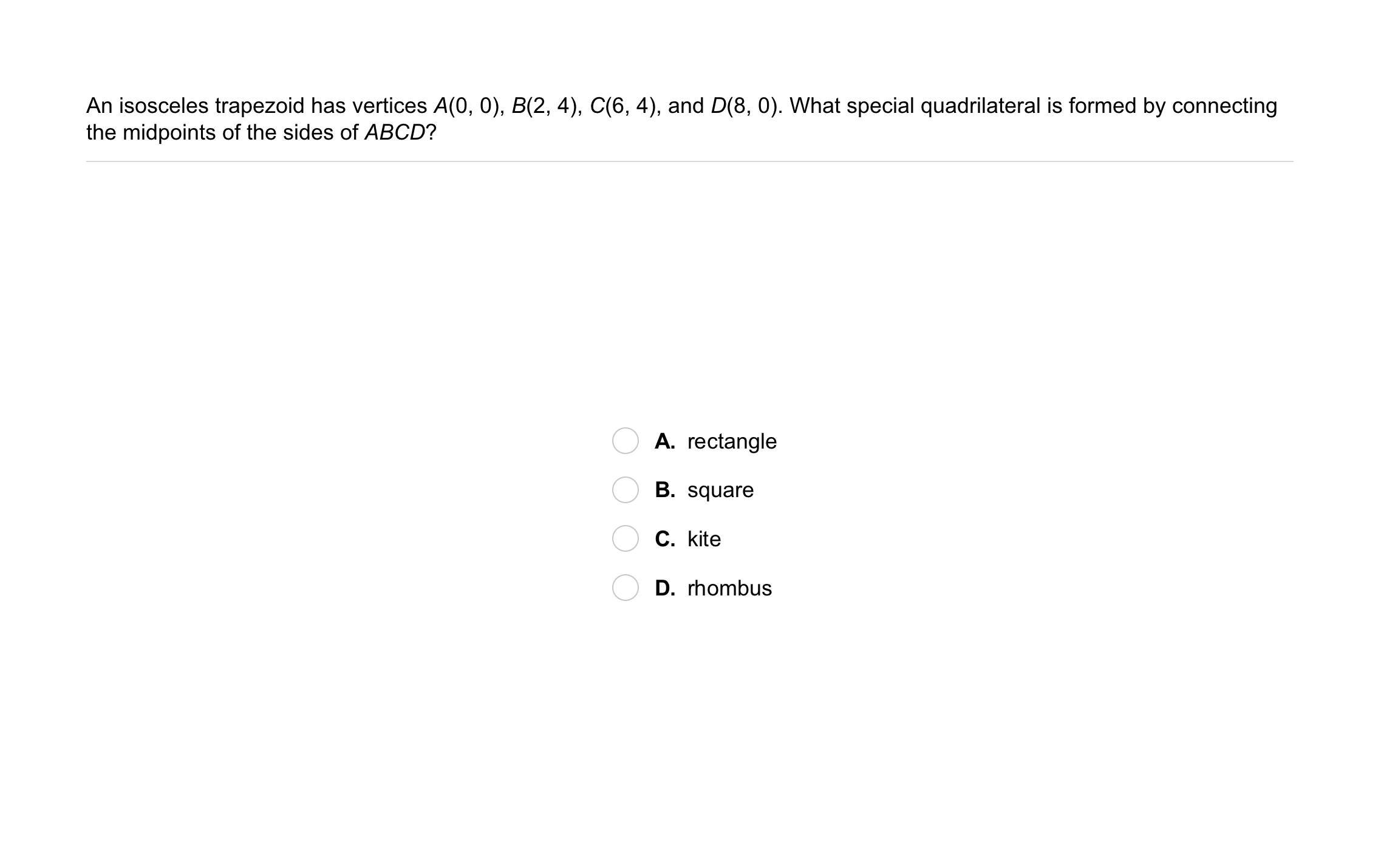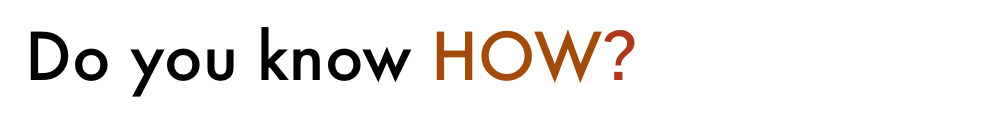Geometry 6-7 Polygons in the Coordinate Plane
starstarstarstarstarstarstarstarstarstar
by Matthew Richardson
| 22 Questions
Note from the author:
A complete formative lesson with embedded slideshow, mini lecture screencasts, checks for understanding, practice items, mixed review, and reflection. I create these assignments to supplement each lesson of Pearson's Common Core Edition Algebra 1, Algebra 2, and Geometry courses. See also mathquest.net and twitter.com/mathquestEDU.
The outlined content above was added from outside of Formative.
The outlined content above was added from outside of Formative.
1
10 pts
Solve It! You and a friend are playing a board game. Players place rubber bands on their own square grid to form different shapes. The object of the game is to guess the vertices of your opponent's shape. How would you place pieces on the grid to complete a right isosceles triangle?
2
10 pts
Solve It! Refer to your sketch in the previous item and justify the placement of each rubber band that you added.The outlined content above was added from outside of Formative.3
3
10 pts
Problem 1 Got It?
A
B
C
The outlined content above was added from outside of Formative.
4
10 pts
Problem 2 Got It? MNPQ has vertices M(0, 1), N(-1, 4), P(2, 5), and Q(3, 2).

a. Is ▱ MNPQ a rectangle? Explain.
5
10 pts
Problem 2 Got It? MNPQ has vertices M(0, 1), N(-1, 4), P(2, 5), and Q(3, 2).

b. Is ▱ MNPQ a square? Explain.
The outlined content above was added from outside of Formative.6
6
10 pts
Problem 3 Got It?
A
B
C
D7
7
10 pts
A
B
C8
8
10 pts
A
B
C
D9
9
10 pts
Writing: Describe how you would determine whether the lengths of the medians from the base angles D and F are congruent.
10
10 pts
Error Analysis: A student says that the quadrilateral with vertices D(1, 2), E(0, 7), F(5, 6), and G(7, 0) is a rhombus because its diagonals are perpendicular. What is the student's error?11
12
13
14
11
5 pts
Review Lesson 6-6: Refer to the isosceles trapezoid shown. Find the value of x.
3/2
2
5/2
5
12
5 pts
Review Lesson 6-6: Refer to the isosceles trapezoid shown. Find m∠1. Enter only a number.
13
5 pts
Review Lesson 6-6: Refer to the isosceles trapezoid shown. Find m∠2. Enter only a number.
14
5 pts
Review Lesson 6-6: Refer to the isosceles trapezoid shown. Find m∠3. Enter only a number.
15
10 pts
Review Lesson 5-3: Find the circumcenter of △ABC.

(3, 2)
(5, 3)
(2, 2)
(2, 3)
16
10 pts
Review Lesson 3-7: Identify the slope of each segment, whose endpoints are given. Not all slopes will be used.
• 0
• 1
• b/(c+d-a)
• -1
• undefined
• (0, a), (-a, 2a)
• (-a, b), (a, b)
• (a, 0), (c+d, b)17
17
10 pts
Vocabulary Review: Identify each item labeled on the coordinate plane. Use the arrow keys to access items, if necessary.
• A
• B
• C
• D
• E
• F
• G
• origin
• x-axis
• y-axis
18
10 pts
Use Your Vocabulary: Complete each statement with the correct word. Use each word only once.
• classification
• classified
• classify
• Trapezoids are __?__ as quadrilaterals.
• Taxonomy is a system of __?__ in biology.
• Schools __?__ children by age.19
20
21
19
5 pts
D
M
S
20
5 pts
D
M
S
21
5 pts
D
M
S
22
10 pts
Reflection: Math Success